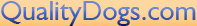A great math lesson:

If:

A B C D E F G H I J K L M N O P Q R S T U V W X Y Z

Is represented as:
1 2 3 4 5 6 7 8 9 10 11 12 13 14 15 16 17 18 19 20 21 22 23 24 25 26

Then:

H-A-R-D-W-O-R- K
8+1+18+4+23+15+18+11 = 98%

and

K-N-O-W-L-E-D-G-E
11+14+15+23+12+5+4+7+5 = 96%
But,

A-T-T-I-T-U-D-E
1+20+20+9+20+21+4+5 = 100%

AND, look how far the love of God will take you

L-O-V-E-O-F-G-O-D
12+15+22+5+15+6+7+15+4 = 101%

Therefore, one can conclude with mathematical certainty that Hard work and
Knowledge will get you close, and Attitude will get you there, but it is

the Love of God that will put you over the top!

Carry each other's burdens and in this way you will fulfill the Law of
Christ.  Galatians 6:2 NIV